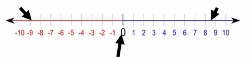# Absolute ValueTo score 700+ on the GMAT, test takers must develop a strategy to answer the Quant questions in 2 minutes and Verbal questions in 1 minute & 20 seconds. Data sufficiency questions can be solved well within the 2-minute mark, most likely in 1 minute and 30 seconds if the conditions and question are rephrased. Not all questions will be required to be rephrased but there are certain conditions where this technique is extremely useful, especially when concepts in Ratio & Proportion, Equations, Inequalities & Divisibility are tested.EquationsThis is the most common question type where rephrasing the conditions might be useful, especially in quadratic equations, and questions that require finding roots. Let us look into a quadratic equation question typeFor the following equations with positive roots, the value of k is greater than one1) 3x^2 +5x + 2k = 02) (x+1)(3x+2) + 2k - 2 = 01) Statement (1) ALONE is sufficient, but statement (2) alone is not sufficient to answer the question asked.2) Statement (2) ALONE is sufficient, but statement (1) alone is not sufficient to answer the question asked.3) BOTH... Categories : Absolute Value, InequalitiesGood news, test takers! You can pass the GMAT! Most of the questions are not that difficult. The difficulty in the test is in taking a mid-level question, (5x2 = 10) and rephrasing it in a more difficult way. (5 men walk into a bar. The bartender gives each man a beer for each of their hands. How many beers does the bartender distribute all together?) There is one exception to the rule, though: Absolute value inequalities.It’s a little tough, but not impossible to figure out absolute value inequalities! We’ll start with absolute value expression’s basic definitions, mathematically and conceptually.  Then we’ll use that basic understanding to solve equations and inequalities including absolute value expressions. Finally, we’ll deal with a concept-based application of the absolute value inequalities on the GMAT. Let’s go! Absolute positive - Everybody knows that |9|=9 and|-9|=9.  What’s so special about those slanted lines? How and why does it neutralize a number?  Those bars are the measure of the distance of the number within the bars and zero. -9 and 9 are both 9 units away from 0. Picture it!... Categories : Absolute Value The GMAT focuses on measuring the candidate’s ability to solve quantitative problems and interpret data. The concept of absolute value inequalities have been known to be the most challenging. With the GMAT being a tightly timed examination, it is crucial that candidates dissect the questions quickly and come up with the correct answers. Let us look at the secrets of mastering absolute value inequalities.An inequality is a comparison between two values or expression where either values or expressions are not equal to one another. Most of the algebraic manipulations that are used in mathematical expressions can be applied to inequalities; however, there are several exceptions listed below that need to be applied. These points may seem trivial; however, the consequences can be severe, and can mislead candidates to the wrong answers.1) When an inequality is being multiplied by a negative value, the direction of the sign must be switched. 2) Unlike expressions, inequalities cannot be squared. This means that a > y will not equal to a^2 > y^2. 3) The square root of both sides cannot be taken to obtain an answer. Inequalities with an absolute value term will typically have two solutions. In order to simplify solving absolute value inequalities on the GMAT examination, candidates should follow these steps: 1)    ... Categories : Absolute ValueAbsolute Values (AVs) questions in GMAT can be a time saver for you if you understand a few rules. Capture the following notes and use it as a reference for your GMAT exam.1. Absolute Value equations are two equations disguised as oneYou can split up any equation involving absolutes into two, and solve for each solution. One will look identical to the given, and the other is found by multiplying the inside by -1. Remember to multiply the entire expression by -1.| (x + 5)/3 | = 11 turns into:(x + 5)/3 = 11, and(x+5)/3 = -11x + 5 = 33x + 5 = -33x = 28 x = -38Note that plugging either x = 28 or x = -38 into the original equation will check out. Also note that solutions for variables within absolute value questions can be negative. What is spit out of the AV cannot be negative, but what goes in can be anything.2. Think of Absolute Values as distances from zeroIf an AV = 15, that means whatever is inside the AV is exactly 15 above or below zero on the number line....

Get F1GMAT's Newsletters (Best in the Industry)

• Ranking Analysis
• Post-MBA Salary Trends
• Post-MBA Job Function & Industry Analysis
• Post-MBA City Review
• MBA Application Essay Tips
• School Specific Essay Tips
• GMAT Preparation Tips